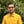Trusted answers to developer questions
Trusted Answers to Developer Questions

Related Tags

python
math
logarithm
communitycreator

# What is math.log() in Python?Harris Amjad

Grokking Modern System Design Interview for Engineers & Managers

Ace your System Design Interview and take your career to the next level. Learn to handle the design of applications like Netflix, Quora, Facebook, Uber, and many more in a 45-min interview. Learn the RESHADED framework for architecting web-scale applications by determining requirements, constraints, and assumptions before diving into a step-by-step design process.

The log() function in Python calculates the logarithm of a number to the base or calculates the natural logarithm of a number if the base is not specified by the user.

The following illustration shows the mathematical representation of the log() function.

Mathematical representation of the log() function

The math module is required in order to use this function.

## Syntax

log(number, base)


## Parameters

This function requires two parameters:

• A numberIt must be greater than 0 for which logarithm is to be calculated.
• The base of the logarithma number which must be greater than one is an optional parameter.

## Return value

log() returns the logarithm of a number to the base sent as a parameter.

Remember that the log() function calculates the natural logarithm of a number if the base is not provided, i.e., log(y,e).

## Code

#Module requiredimport math#number without a baseprint "log(20) : ", math.log(20)#number with a baseprint "log(2,2) : ", math.log(2,2)

RELATED TAGS

python
math
logarithm
communitycreator

CONTRIBUTORHarris Amjad

Grokking Modern System Design Interview for Engineers & Managers

Ace your System Design Interview and take your career to the next level. Learn to handle the design of applications like Netflix, Quora, Facebook, Uber, and many more in a 45-min interview. Learn the RESHADED framework for architecting web-scale applications by determining requirements, constraints, and assumptions before diving into a step-by-step design process.

Keep Exploring

Learn in-demand tech skills in half the time